# NCERT Solutions - Determinants, Exercise 4.1 JEE Notes | EduRev

## JEE : NCERT Solutions - Determinants, Exercise 4.1 JEE Notes | EduRev

The document NCERT Solutions - Determinants, Exercise 4.1 JEE Notes | EduRev is a part of the JEE Course Mathematics (Maths) Class 12.
All you need of JEE at this link: JEE

Determinants

Exercise 4.1
Question 1: Evaluate the determinants in Exercises 1 and 2.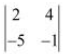= 2(−1) − 4(−5) = − 2 + 20 = 18

Question 2: Evaluate the determinants in Exercises 1 and 2.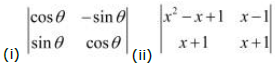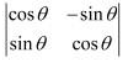(i) = (cos θ)(cos θ) − (−sin θ)(sin θ) = cos2 θ+ sin2 θ = 1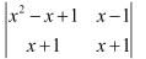(ii) = (x2 − x + 1)(x + 1) − (x − 1)(x + 1)
= x3 − x2 + x + x2 − x + 1 − (x2 − 1)
= x+ 1 − x2 + 1
= x− x2 + 2

Question 3: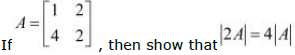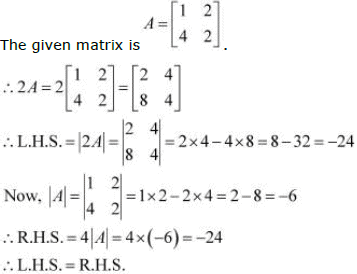.

Question 4: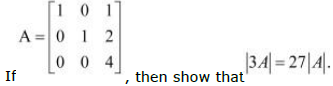The given matrix is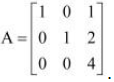.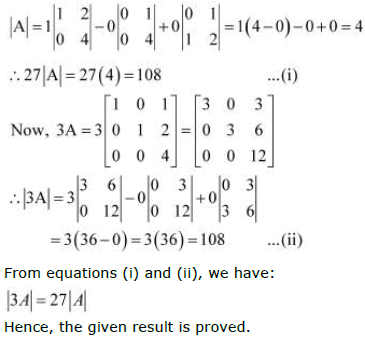Question 5: Evaluate the determinants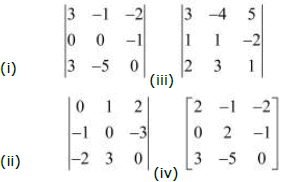(i) Let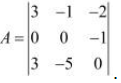It can be observed that in the second row, two entries are zero. Thus, we expand along the second row for easier calculation.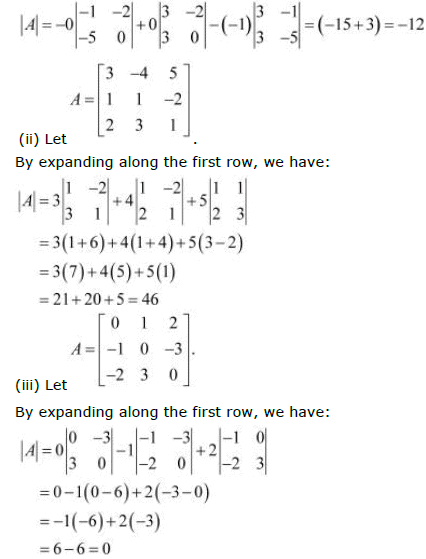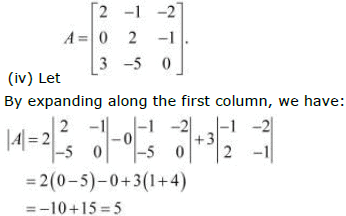Question 6:
If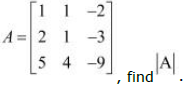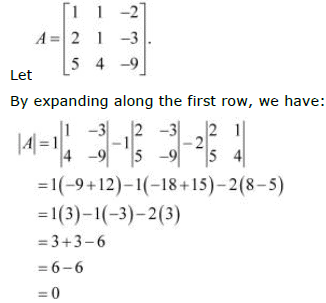Question 7: Find values of x, if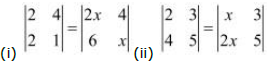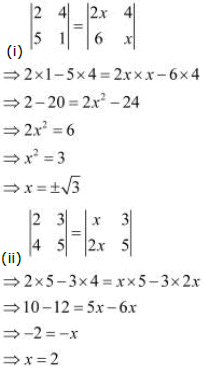Question 8:
If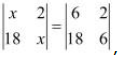, then x is equal to

(A) 6

(B) ±6

(C) −6

(D) 0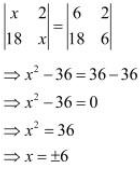Hence, the correct answer is B.

Offer running on EduRev: Apply code STAYHOME200 to get INR 200 off on our premium plan EduRev Infinity!

## Mathematics (Maths) Class 12

209 videos|222 docs|124 tests

,

,

,

,

,

,

,

,

,

,

,

,

,

,

,

,

,

,

,

,

,

,

,

,

;